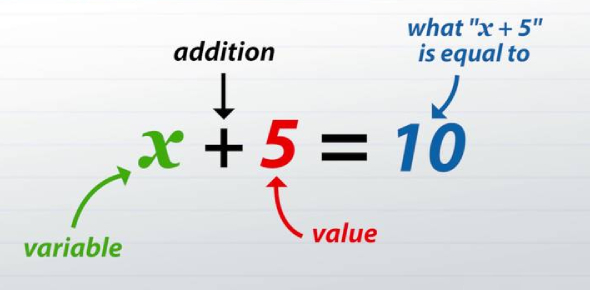# Combining Like Terms And Equation Quiz!

10 Questions | Total Attempts: 145Settings.

• 1.
Solve: x + 8 = 15
• A.

X = 7

• B.

X = -7

• C.

X = 23

• D.

X = -23

• 2.
Solve: y - 3 = -5
• A.

Y = 8

• B.

Y = -8

• C.

Y = 2

• D.

Y = -2

• 3.
Solve: -2x = 88
• A.

X = 44

• B.

X = -44

• C.

X = 86

• D.

X = 90

• 4.
Solve: r/5 = -9
• A.

R = -14

• B.

R = -4

• C.

R = -45

• D.

R = 45

• 5.
Simplify: 3x + 4 - 8x
• A.

-5x + 4

• B.

11x + 4

• C.

15x

• D.

-x

• 6.
Simplify: -6(x + 5) + 2x
• A.

-4x + 30

• B.

-4x + 5

• C.

8x + 5

• D.

-4x - 30

• 7.
Simplify: 7p - 5m + 6p + 3m
• A.

13p + 8m

• B.

13p - 2m

• C.

P + 8m

• D.

21pm

• 8.
What operation should you do first in order to solve the following equation: -9 + x = 43
• A.

• B.

Subtract 9

• C.

Multiply by -9

• D.

Divide by -9

• 9.
What operation should you do first in order to solve the following equation: t/5 = 85
• A.

• B.

Subtract 5

• C.

Multiply by 5

• D.

Divide by 5

• 10.
To combine like terms, we should...
• A.

• B.

Multiply & divide them

• C.Back to top